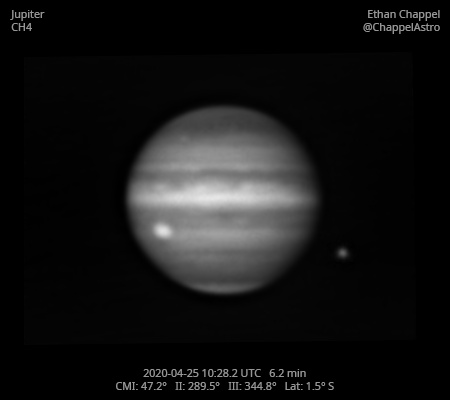# Jupiter 2020-04-25 10:28 UTC

CM1: 47.20°

CM2: 289.50°

CM3: 344.80°

CLat: -1.50°

Description

Compared to previous mornings, seeing was closer to good. Unfortunately for me, I set up the C8 before going to bed in anticipation of strong winds, but it ended up being calm enough for the C14. Nevertheless, I was happy to the good conditions while imaging Jupiter.

Notes:

• The Great Red Spot is rotating into view.
• A white barge in the SEB is on the central meridian.
• Callisto is approaching occultation by Jupiter.

Equipment

ZWO ASI290MM

Celestron EdgeHD 8

Logs
```FireCapture v2.6  Settings
------------------------------------
Observer=Ethan Chappel
Camera=ZWO ASI290MM
Filter=CH4
Profile=Jupiter
Diameter=39.92"
Magnitude=-2.31
CMI=47.6° CMII=289.9° CMIII=345.2°  (during mid of capture)
FocalLength=1550mm (F/4)
Resolution=0.39"
Filename=2020-04-25-1029_8-EC-CH4.ser
Date=2020_04_25
Start=10_28_21.448
Mid=10_29_51.458
End=10_31_21.468
Start(UT)=10_28_21.448
Mid(UT)=10_29_51.458
End(UT)=10_31_21.468
Duration=180.020s
Date_format=yyyy_MM_dd
Time_format=HH_mm_ss
LT=UT -6h
Frames captured=3001
File type=SER
Binning=no
Bit depth=8bit
Debayer=no
ROI=264x196
ROI(Offset)=32x0
FPS (avg.)=16
Shutter=60.00ms
Gain=400 (66%)
AutoHisto=75 (off)
HighSpeed=off
AutoExposure=off
Brightness=1 (off)
Gamma=50 (off)
USBTraffic=40 (off)
SoftwareGain=10 (off)
FPS=100 (off)
AutoGain=off
Histogramm(min)=1
Histogramm(max)=116
Histogramm=45%
Noise(avg.deviation)=4.15
AutoAlign=false
PreFilter=none
Limit=180 Seconds
Sensor temperature=26.0°C
FireCapture v2.6  Settings
------------------------------------
Observer=Ethan Chappel
Camera=ZWO ASI290MM
Filter=CH4
Profile=Jupiter
Diameter=39.92"
Magnitude=-2.31
CMI=45.6° CMII=287.9° CMIII=343.3°  (during mid of capture)
FocalLength=1550mm (F/4)
Resolution=0.39"
Filename=2020-04-25-1026_6-EC-CH4.ser
Date=2020_04_25
Start=10_25_08.932
Mid=10_26_38.955
End=10_28_08.978
Start(UT)=10_25_08.932
Mid(UT)=10_26_38.955
End(UT)=10_28_08.978
Duration=180.046s
Date_format=yyyy_MM_dd
Time_format=HH_mm_ss
LT=UT -6h
Frames captured=3001
File type=SER
Binning=no
Bit depth=8bit
Debayer=no
ROI=264x196
ROI(Offset)=32x0
FPS (avg.)=16
Shutter=60.00ms
Gain=400 (66%)
AutoHisto=75 (off)
HighSpeed=off
AutoExposure=off
Brightness=1 (off)
Gamma=50 (off)
USBTraffic=40 (off)
SoftwareGain=10 (off)
FPS=100 (off)
AutoGain=off
Histogramm(min)=0
Histogramm(max)=105
Histogramm=41%
Noise(avg.deviation)=4.12
AutoAlign=false
PreFilter=none
Limit=180 Seconds
Sensor temperature=26.7°C
```